# sian.mat

•### Rsin(Nx+a)

Activity

sian.mat

•### Wave Equation for PowerPoint Example

Activity

sian.mat

•### Wave Equation

Activity

sian.mat

•### Vector representation of phasors

Activity

sian.mat

•### Differentiation of e^x

Activity

sian.mat

•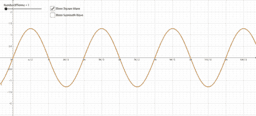### Fourier Demonstration

Activity

sian.mat

•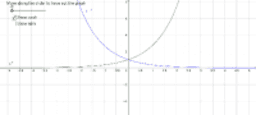### Hyperbolic Graphs

Activity

sian.mat

•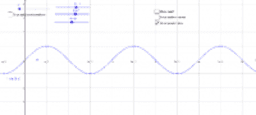Activity

sian.mat

•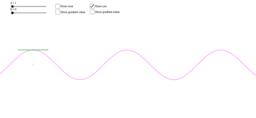### Demonstration of sin and cos differential

Activity

sian.mat

•Activity

sian.mat

•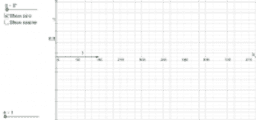### sine and cos

Activity

sian.mat

•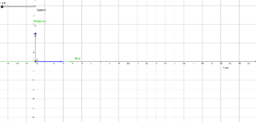### AC current as phasor

Activity

sian.mat

•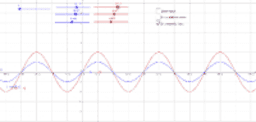Activity

sian.mat

•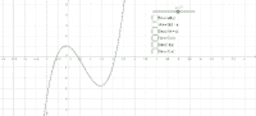### Graph Transformations

Activity

sian.mat

•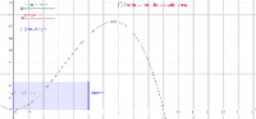### Comparing Integration to Area Under Graph

Activity

sian.mat

•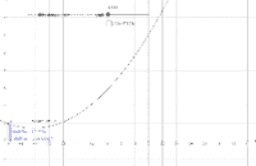### Demonstration of how integration gives us area under a curve

Activity

sian.mat

•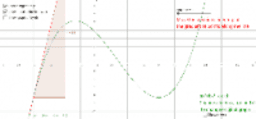Activity

sian.mat

•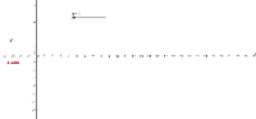### Differentiation of e^x

Activity

sian.mat

•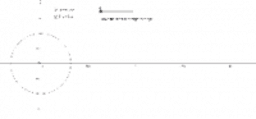Activity

sian.mat